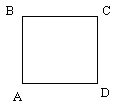Email us to get an instant 20% discount on highly effective K-12 Math & English kwizNET Programs!

Online Quiz (WorksheetABCD)

Questions Per Quiz = 2 4 6 8 10

Grade 5 - Mathematics7.12 Rhombus

 The rhombus is a quadrilateral with all sides equal in length. Rhombus is a parallelogram with four sides of the same length. A square is a special kind of rhombus with four sides of same length and four right angles. The rhombus is also called a diamond.The diagonals of a rhombus bisect perpendicularly.Directions: Answer the following questions. Also draw a rhombus and write its properties.
 Q 1: A __ is a special kind of rhombus with four sides of same length and four right angles.squareparallelogramrectangle Q 2: The diagonals of a rhombus divide each other in half where they intersect. The angle formed is __obtuse angleright angleacute angle Q 3: A parallelogram with four sides of the same length is calledSquareRhombusRectangle Q 4: How many sides does a rhombus have?648 Question 5: This question is available to subscribers only! Question 6: This question is available to subscribers only!

Subscription to kwizNET Learning System offers the following benefits:

• Unrestricted access to grade appropriate lessons, quizzes, & printable worksheets
• Instant scoring of online quizzes
• Progress tracking and award certificates to keep your student motivated
• Unlimited practice with auto-generated 'WIZ MATH' quizzes